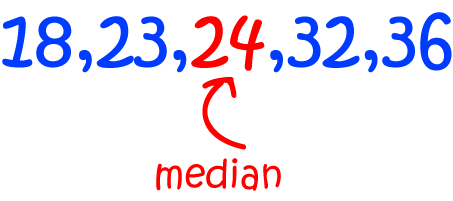# Definition of MedianThe median of a list of numbers is the middle value when the numbers are placed in increasing or decreasing order.

To find the median of a list of numbers:

1. Place the numbers in increasing order.
2. Count the numbers.
3. If there are an odd number of numbers, the middle one is the median.
4. If there an even number of numbers, take the average of the two middle numbers to give the median.

For example, to find the median of the list $\{3,1,5,7,2\}$:

1. Place the numbers in increasing order: $\{1,2,3,5,7\}$
2. Count the numbers: there are 5, which is odd.
3. The middle one is the median: the median is the third number, which is $3$.
So, the median is $3$.

For example, to find the median of the list $\{3,1,5,7,2,4\}$:

1. Place the numbers in increasing order: $\{1,2,3,4,5,7\}$
2. Count the numbers: there are 6, which is even.
3. The average of the two middle numbers is the median: the two middle numbers are $3$ and $4$. Their average is $\dfrac{3 + 4}{2} = 3.5$.
So, the median is $3.5$.

### Description

The aim of this dictionary is to provide definitions to common mathematical terms. Students learn a new math skill every week at school, sometimes just before they start a new skill, if they want to look at what a specific term means, this is where this dictionary will become handy and a go-to guide for a student.

### Audience

Year 1 to Year 12 students

### Learning Objectives

Learn common math terms starting with letter M

Author: Subject Coach
You must be logged in as Student to ask a Question.